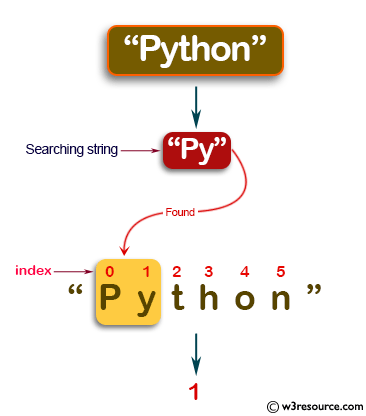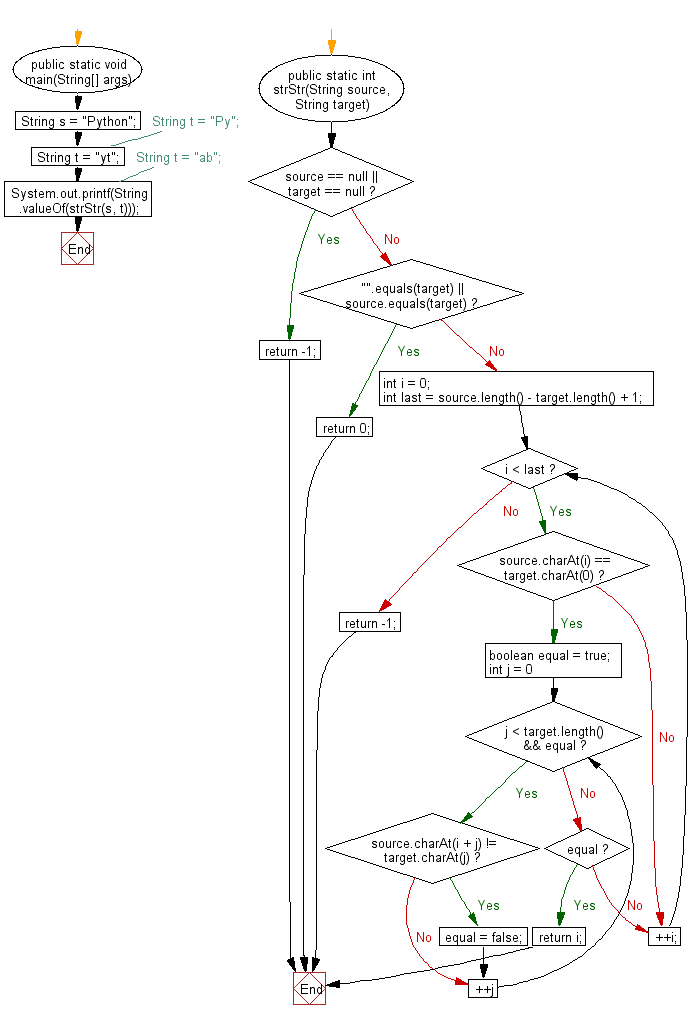﻿ Java: Get the first occurrence of a string within a string# Java Exercises: Get the first occurrence of a string within a given string

## Java Basic: Exercise-118 with Solution

Write a Java program to get the first occurrence (Position starts from 0.) of a string within a given string.

Pictorial Presentation:Sample Solution:

Java Code:

``````import java.util.Scanner;
public class Main {
public static void main(String[] args) {
String s = "Python";
//String t = "Py";
String t = "yt";
// String t = "ab";
System.out.printf(String.valueOf(strStr(s, t)));
}
public static int strStr(String source, String target) {
if (source == null || target == null) {
return -1;
}
if ("".equals(target) || source.equals(target)) {
return 0;
}
int i = 0;
int last = source.length() - target.length() + 1;
while (i < last) {
if (source.charAt(i) == target.charAt(0)) {
boolean equal = true;
for (int j = 0; j < target.length() && equal; ++j) {
if (source.charAt(i + j) != target.charAt(j)) {
equal = false;
}
}
if (equal) {
return i;
}
}
++i;
}
return -1;
}
}
```
```

Sample Output:

```1
```

Flowchart:Java Code Editor:

What is the difficulty level of this exercise?

Test your Programming skills with w3resource's quiz.

﻿

## Java: Tips of the Day

countOccurrences

Counts the occurrences of a value in an array.

Use Arrays.stream().filter().count() to count total number of values that equals the specified value.

```public static long countOccurrences(int[] numbers, int value) {
return Arrays.stream(numbers)
.filter(number -> number == value)
.count();
}
```

Ref: https://bit.ly/3kCAgLb